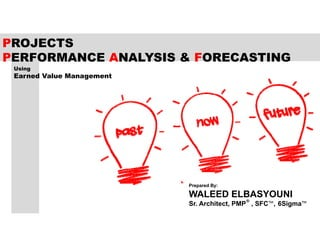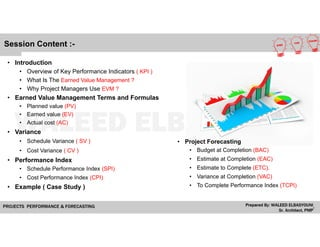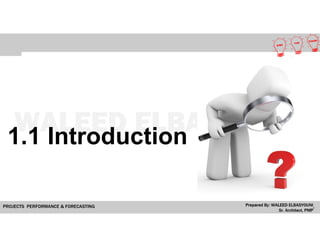Se está descargando tu SlideShare. ×

# Projects Performance Analysis & Forecasting

Anuncio
Anuncio
Anuncio
Anuncio
Anuncio
Anuncio
Anuncio
Anuncio
Anuncio
Anuncio
AnuncioCargando en…3
×

1 de 25 Anuncio

# Projects Performance Analysis & Forecasting

Introduction
Overview of Key Performance Indicators ( KPI )
What Is The Earned Value Management ?
Why Project Managers Use EVM ?
Earned Value Management Terms and Formulas
Planned value (PV)
Earned value (EV)
Actual cost (AC)
Variance
Schedule Variance ( SV )
Cost Variance ( CV )
Performance Index
Schedule Performance Index (SPI)
Cost Performance Index (CPI)
Example ( Case Study )
Project Forecasting
Budget at Completion (BAC)
Estimate at Completion (EAC)
Estimate to Complete (ETC).
Variance at Completion (VAC)
To Complete Performance Index (TCPI)

Introduction
Overview of Key Performance Indicators ( KPI )
What Is The Earned Value Management ?
Why Project Managers Use EVM ?
Earned Value Management Terms and Formulas
Planned value (PV)
Earned value (EV)
Actual cost (AC)
Variance
Schedule Variance ( SV )
Cost Variance ( CV )
Performance Index
Schedule Performance Index (SPI)
Cost Performance Index (CPI)
Example ( Case Study )
Project Forecasting
Budget at Completion (BAC)
Estimate at Completion (EAC)
Estimate to Complete (ETC).
Variance at Completion (VAC)
To Complete Performance Index (TCPI)

Anuncio
Anuncio

### Projects Performance Analysis & Forecasting

1. 1. PROJECTS PERFORMANCE ANALYSIS & FORECASTING ® Prepared By: WALEED ELBASYOUNI Sr. Architect, PMP , SFC™, 6Sigma™ Using Earned Value Management
2. 2. Session Content :- • Introduction • Overview of Key Performance Indicators ( KPI ) • What Is The Earned Value Management ? • Why Project Managers Use EVM ? • Earned Value Management Terms and Formulas • Planned value (PV) • Earned value (EV) • Actual cost (AC) • Variance • Schedule Variance ( SV ) • Cost Variance ( CV ) • Performance Index • Schedule Performance Index (SPI) • Cost Performance Index (CPI) • Example ( Case Study ) • Project Forecasting • Budget at Completion (BAC) • Estimate at Completion (EAC) • Estimate to Complete (ETC). • Variance at Completion (VAC) • To Complete Performance Index (TCPI)
3. 3. 1.1 Introduction
4. 4. 1.1 Introduction Overview of Key Performance Indicators ( KPI ) • Key Performance Indicators ( KPI ) are a Set Of Quantifiable Measures That a Company Uses To Gauge Its Performance Over Time. • These Metrics Are Used To Determine a Company's Progress In Achieving Its Strategic and Operational Goals, And Also To Compare A Company's Performance Against Other Businesses Within Its Industry.
5. 5. What Is The Earned Value Management ? • Earned Value Management Is a Project Management Technique For Measuring Project Performance And Progress. • Earned Value Is An Approach Where You Monitor The Project Plan, Actual Work, And Work-completed Value To See If a Project Is On Track. • Earned Value Shows How Much Of The Budget And Time Should Have Been Spent, With Regard To The Amount Of Work Done So Far. Why Project Managers Use EVM ? • Project Managers Use EVM To Assess The Schedule and Cost Performance Of a Project To Know Exactly Whether The Project Is: (Ahead Of / On / Behind Schedule) - ( Under / On / Over Budget ) 1.1 Introduction
6. 6. 1.2 Earned Value Management Terms and Formulas
7. 7. Planned value Earned value Actual Cost PV EV AC 1.2 Earned Value Management Terms and Formulas  Any Project Manager Use Earned Value Management To Assess The Schedule and Cost Performance Of a Project He Should Based On Three Terms. EVMEVM PVPV EVEVACAC
8. 8. Budgeted Cost For Work Scheduled (BCWS) Planned valuePV • The Approved Budget For The Work Scheduled To Be Completed By a Specified Date. • Also Referred To As The Budgeted Cost Of Work Scheduled (BCWS). 1.2 Earned Value Management Terms and Formulas
9. 9. Budgeted Cost For Work Performed (BCWP) Earned valueEV • The Approved Budget For The Work Actually Completed By The Specified Date. • Also Referred To As The Budgeted Cost Of Work Performed (BCWP). 1.2 Earned Value Management Terms and Formulas
10. 10. • The Costs Actually Incurred For The Work Completed By The Specified Date. • Also Referred To As The Actual Cost Of Work Performed (ACWP). Actual cost for work performed (ACWP) Actual CostAC 1.2 Earned Value Management Terms and Formulas
11. 11. 1.3 Project Variance & Performance Index
12. 12. Project Variance Schedule Variance Cost Variance SV CV EV – PV EV – AC Project Variance According To Earned Value Management Three Terms We Can Define The Project Variance :- • Planned Value (PV) • Earned Value (EV) • Actual Cost (AC) 1.3 Project Variance & Performance Index
13. 13. SV < 0 Behind Schedule SV = 0 On Schedule SV > 0 Ahead of Schedule Planned Value (PV) - Earned value (EV) - Actual Cost (AC) Schedule Variance (SV) = EV – PV 1.3 Project Variance & Performance Index
14. 14. CV < 0 Over Budget CV = 0 On Budget CV > 0 Under Budget Planned Value (PV) - Earned value (EV) - Actual Cost (AC) Cost Variance (CV) = EV – AC 1.3 Project Variance & Performance Index
15. 15. Project Performance Index Schedule Performance Index Cost Performance Index SPI CPI EV / PV EV / AC Performance Index According To Earned Value Management Three Terms We Can Define The Project Performance Index :- • Planned Value (PV) • Earned Value (EV) • Actual Cost (AC) 1.3 Project Variance & Performance Index
16. 16. SPI < 1 Behind Schedule SPI = 1 On Schedule SPI > 1 Ahead of Schedule Schedule Performance Index (SPI) = EV / PV Planned Value (PV) - Earned value (EV) - Actual Cost (AC) 1.3 Project Variance & Performance Index
17. 17. Cost Performance Index (CPI) = EV / AC Planned Value (PV) - Earned value (EV) - Actual Cost (AC) CPI < 1 Over Budget CPI = 1 On Budget CPI > 1 Under Budget 1.3 Project Variance & Performance Index
18. 18.  Planned Value (PV)  Earned value (EV)  Actual Cost (AC)  Schedule Variance (SV)  Cost Variance (CV)  Schedule Performance Index (SPI)  Cost Performance Index (CPI) 1.3 Project Variance & Performance Index Quantity Cost | Unit Budget 100 500 S.R Actual 120 600 S.R Example ( Case Study ) • This Schedule Shows Some Information About Budgeted And Actual Cost To (XYZ) Construction Project , According To EVM Techniques Calculate The Following Terms Then Define The Project Status (Schedule & Budget) ? • PV = 500 x 100 = 50.000 S.R • EV = 500 x 120 = 60.000 S.R • AC = 600 x 120 = 72.000 S.R • SV = EV – PV = 60.000 – 50.000 = 10.000 S.R • CV = EV – AC = 60.000 – 72.000 = -12.000 S.R • SPI = EV / PV = 60.000 / 50.000 = 1.2 • CPI = EV / AC = 60.000 / 72.000 = 0.83 The Project (XYZ ) Status at this Time • According SV & SPI The Project Ahead Of Schedule. • According CV & CPI The Project Over Budget.
19. 19. 1.4 Project Forecasting
20. 20. 1.4 Project Forecasting Project Forecasting • Consists Of Taking The Project Status Information And Extrapolating The Current Project Performance To The End Of The Project and How The Future Will Turn Out Based On Evidence Or Assumptions. • The Purpose Of Forecasting Is To Give Managers Insight Into How Profitable Projects Are Likely To Be In The Future.
21. 21. 1.4 Project Forecasting Forecasting Terms :- • Budget at Completion (BAC) • Estimate at Completion (EAC) • Estimate to Complete (ETC)  Considering The Same Budgeted Rate  Considering CPI  Considering CPI and SPI • Variance At Completion (VAC) • To Complete Performance Index (TCPI)
22. 22. 1.4 Project Forecasting • Estimate To Complete (ETC) Is The Estimated Cost Required To Complete The Remainder Of The Project There Are Various Methods To Calculate The (ETC):-  Considering The Same Budgeted Rate EAC = AC + (BAC – EV)  Considering CPI EAC = BAC / CPI  Considering CPI and SPI EAC = AC + [ (BAC – EV) / (CPI x SPI) ] • Budget At Completion (BAC) Is The Total Budget Allocated To The Project • Estimate At Completion (EAC) Is The Expected Total Cost Of A Schedule Activity Component, EAC Is Equal To The Actual Cost Of Work Performed (ACWP) + The Estimate To Complete (ETC) For All Of The Remaining Work.
23. 23. 1.4 Project Forecasting • Variance At Completion (VAC) Is The Difference Between The Budget At Completion(BAC) And The Estimate At Completion (EAC) VAC = BAC – EAC • To Complete Performance Index (TCPI) It’s The Cost Performance That Must Be Achieved On The Remaining Work To Meet A Specified Goal Like BAC Or EAC, It Represents The Ratio Between Remaining Works And Remaining Funds. TCPI = (BAC- EV) / (BAC – AC) Or TCPI = (BAC-EV) / (EAC – AC) From the Previous Example • PV = 500 x 100 = 50.000 S.R • EV = 500 x 120 = 60.000 S.R • AC = 600 x 120 = 72.000 S.R • Assumed BAC = 500.000 S.R TCPI = (BAC-EV) --------------------- (BAC – AC) TCPI = 500.000 - 60.000 ----------------------- 500.000 – 72.000 TCPI = 1.028 TCPI = 440.000 -------------- 428.000